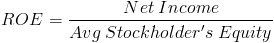# Return on Equity (ROE)The formula for return on equity, sometimes abbreviated as ROE, is a company's net income divided by its average stockholder's equity. The numerator of the return on equity formula, net income, can be found on a company's income statement.

## Average Stockholder's Equity in the ROE Formula

The denominator of the return on equity formula, average stockholder's equity, can be found on a company's balance sheet. Stockholder's equity is a company's assets minus its liabilities. When calculating the return on equity, the stockholder's equity should be averaged based on the time being evaluated. For example, if an investor is calculating the return on equity for 2012, then the beginning and ending stockholder's equity should be used.

Stockholder's equity is also referred to as net assets.

## ROE Formula vs. Return on Assets Formula

The difference between return on equity and return on assets can be found in the denominators of each formula. For return on assets, the denominator is average total assets and for the return on equity formula, the denominator is average stockholder's equity. Both of these variables can be found on a company's balance sheet.

Assets shown on a balance sheet is stockholder's equity plus liabilities. Therefore, the return on equity formula is the same as return on assets except that it does not include liabilities.

## Use of ROE Formula

The return on equity can be used internally by a company or can be used by an investor to evaluate how well the company is turning a profit relative to its stockholder's equity.

## Alternative ROE Formula

The return on equity can also be calculated by multiplying Profit Margin x Asset Turnover x Equity Multiplier. See Return on Equity DuPont for further explanation.

New to Finance?